# Goodness of Fit and Model Comparison¶

## Goodness of fit¶

It is often that we we need to know how well our model fits our data. While in linear, Gaussian regimes and under certain regularity conditions, the reduced $$\chi^2$$ provides a measure of fit quality, most of the time it is unreliable and incorrect to use. For more on this, read The Do’s and Don’ts of reduced chi2.

Instead, we can almost always use the bootstrap method to estimate the quality of an MLE analysis. In 3ML, we can do this with the quite simply after a fit.

:

%matplotlib inline
import matplotlib.pyplot as plt
from jupyterthemes import jtplot

jtplot.style(context='talk', fscale=1, ticks=True, grid=False)
plt.style.use('mike')

import scipy.stats as stats
import numpy as np

from threeML import *

import warnings
warnings.simplefilter('ignore')


Let’s go back to simulations. We will simulate a straight line.

:

gen_function = Line(a=0,b=1)

x = np.linspace(0, 2, 50)

xyl_generator = XYLike.from_function("sim_data", function = gen_function,
x = x,
yerr = 0.3 * gen_function(x))

y = xyl_generator.y
y_err = xyl_generator.yerr

xyl_generator.plot();


Using Gaussian statistic (equivalent to chi^2) with the provided errors.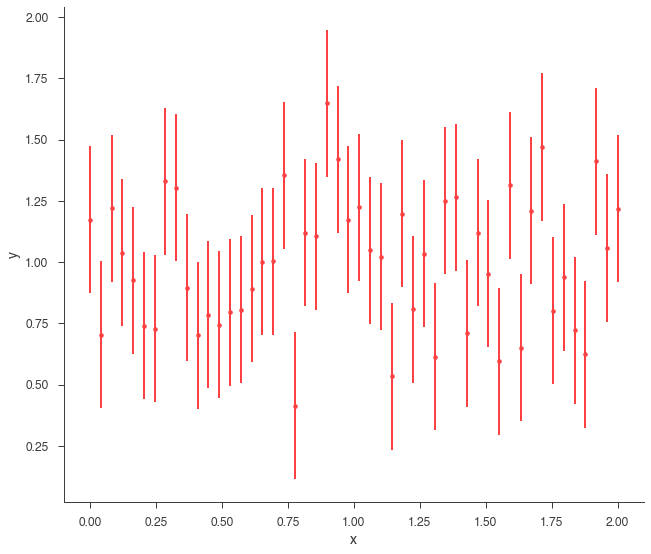So, now we simply need to fit the data.

:

fit_function = Line()

xyl = XYLike("data", x, y, y_err)

datalist = DataList(xyl)

model = Model(PointSource('xyl',0,0,spectral_shape=fit_function))

jl = JointLikelihood(model, datalist)

jl.fit();

xyl.plot();


Using Gaussian statistic (equivalent to chi^2) with the provided errors.
Best fit values:


result unit
parameter
xyl.spectrum.main.Line.a (3 +/- 7) x 10^-2 1 / (cm2 keV2 s)
xyl.spectrum.main.Line.b (9.6 +/- 0.8) x 10^-1 1 / (cm2 keV s)

Correlation matrix:


 1 -0.86 -0.86 1

Values of -log(likelihood) at the minimum:


-log(likelihood)
data 21.023393
total 21.023393

Values of statistical measures:


statistical measures
AIC 46.302106
BIC 49.870833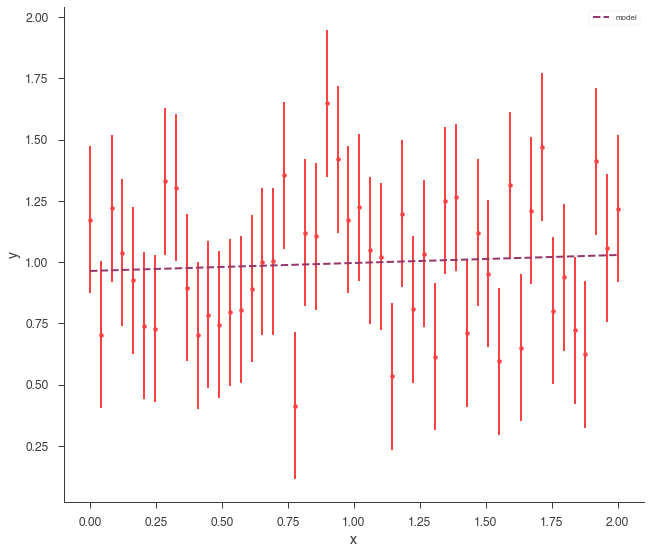Now that the data are fit, we can assess the goodness of fit via simulating synthetic data sets and seeing how often these datasets have a similar likelihood. To do this, pass the JointLikelihood object to the GoodnessOfFit class.

:

gof_obj = GoodnessOfFit(jl)


Now we will monte carlo some datasets. This can be computationally expensive, so we will use 3ML’s built in context manager for accessing ipython clusters. If we have a profile that is connected to a super computer, then we can simulate and fit all the datasets very quickly.

:

with parallel_computation():
gof, data_frame, like_data_frame = gof_obj.by_mc(n_iterations=8000)

Starting ipyparallel cluster with this command line:
/Users/jburgess/.environs/3ml/bin/ipcluster start
Waiting for connection file: ~/.ipython/profile_default/security/ipcontroller-client.json
12 engines are active


Shutting down ipcluster...


Three things are returned, the GOF for each plugin (in our case one) as well as the total GOF, a data frame with the fitted values for each synthetic dataset, and the likelihoods for all the fits. We can see that the data have a reasonable GOF:

:

gof

:

OrderedDict([('total', 0.712625), ('data', 0.712625)])


## Likelihood Ratio Tests¶

An essential part of MLE analysis is the likelihood ratio test (LRT) for comparing models. For nested models (those where one is a special case of the other), Wilks’ theorem posits that the LRT is $$\chi^2$$ distributed, and thus the null model can be rejected with a probability read from a $$\chi^2$$ table.

In a perfect world, this would always hold, but there are many regualrity conditions on Wilks’ theorem that are often violated in astromonical data. For a review, see Protassov et al and keep it close at heart whenever wanting to use the LRT.

For these reasons, in 3ML we provide a method for computing the LRT via profiling the null model via bootstrap samples. This is valid for nested models and avoids the dangers of asymmptotics and parameters defined on the extreme boundries of their distributions (spectral line normalizations, extra spectral components, etc.). This method does not avoid other problems which may arise from systmatics present in the data. As with any analysis, it is important to doubt and try and prove the result wrong as well as understanding the data/instrument.

Let’s start by simulating some data from a power law with an exponential cutoff on top of a background.

:

energies = np.logspace(1,3,51)

low_edge = energies[:-1]
high_edge = energies[1:]

# get a blackbody source function
source_function = Cutoff_powerlaw(K=1,index=-1,xc=300,piv=100)

# power law background function
background_function = Powerlaw(K=1,index=-2., piv=100.)

spectrum_generator = SpectrumLike.from_function('fake',
source_function=source_function,
background_function=background_function,
energy_min=low_edge,
energy_max=high_edge)


:

spectrum_generator.view_count_spectrum();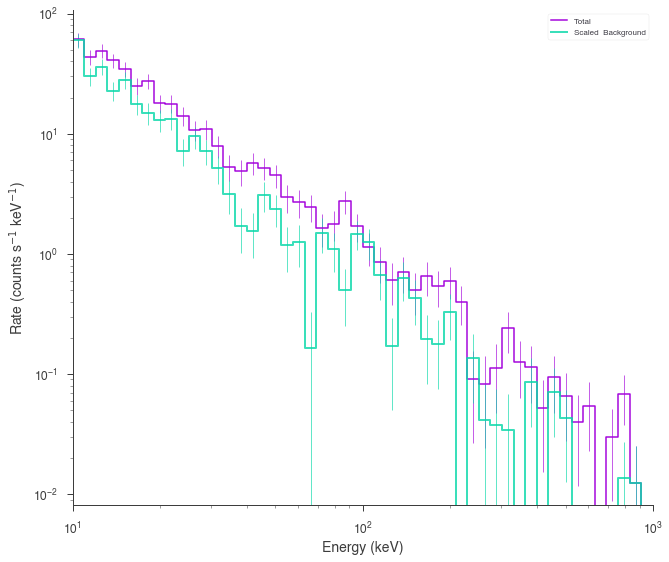We simulated a weak cutoff powerlaw. But if this was real data, we wouldn’t know that there was a cutoff. So we would fit both a power law (the null model) and a cutoff power law (the alternative model).

Let’s setup two models to fit the data via MLE in the standard 3ML way.

:

powerlaw = Powerlaw(piv=100)
cutoff_powerlaw = Cutoff_powerlaw(piv=100)

ps_powerlaw = PointSource('test_pl', 0, 0 , spectral_shape=powerlaw)
ps_cutoff_powerlaw = PointSource('test_cpl', 0, 0, spectral_shape=cutoff_powerlaw)

model_null = Model(ps_powerlaw)
model_alternative = Model(ps_cutoff_powerlaw)

:

datalist = DataList(spectrum_generator)

:

jl_null = JointLikelihood(model_null,datalist)
jl_null.fit();

Best fit values:


result unit
parameter
test_pl.spectrum.main.Powerlaw.K (5.7 -0.6 +0.7) x 10^-1 1 / (cm2 keV s)
test_pl.spectrum.main.Powerlaw.index -1.55 +/- 0.07

Correlation matrix:


 1 0.32 0.32 1

Values of -log(likelihood) at the minimum:


-log(likelihood)
fake 207.424608
total 207.424608

Values of statistical measures:


statistical measures
AIC 419.104535
BIC 422.673262
:

jl_alternative = JointLikelihood(model_alternative,datalist)
jl_alternative.fit();

Best fit values:


result unit
parameter
test_cpl.spectrum.main.Cutoff_powerlaw.K (8.6 -1.8 +2.2) x 10^-1 1 / (cm2 keV s)
test_cpl.spectrum.main.Cutoff_powerlaw.index -1.28 +/- 0.16
test_cpl.spectrum.main.Cutoff_powerlaw.xc (5.0 -2.2 +4) x 10^2 keV

Correlation matrix:


 1 0.83 -0.9 0.83 1 -0.85 -0.9 -0.85 1

Values of -log(likelihood) at the minimum:


-log(likelihood)
fake 205.630008
total 205.630008

Values of statistical measures:


statistical measures
AIC 417.781755
BIC 422.996084

Ok, we now have our log(likelihoods) from each model. If we took Wilks’ theorem to heart, then we would compute:

$\Lambda(x)=\frac{\sup\{\,\mathcal L(\theta\mid x):\theta\in\Theta_0\,\}}{\sup\{\,\mathcal L(\theta\mid x) : \theta\in\Theta\,\}}$

or $$-2 \log(\Lambda)$$ which would be $$\chi^2_{\nu}$$ distributed where $$\nu$$ is the number of extra parameters in the alternative model. In our case:

:

# calculate the test statistic
TS = 2*(jl_null.results.get_statistic_frame()['-log(likelihood)']['total'] - jl_alternative.results.get_statistic_frame()['-log(likelihood)']['total'])

print('null hyp. prob.: %f' %stats.chi2.pdf(TS,1))


null hyp. prob.: 0.034997


But lets check this by simulating the null distribution.

We create a LRT object by passing the null model and the alternative model (in that order).

:

lrt = LikelihoodRatioTest(jl_null,jl_alternative)


Now we MC synthetic datasets again.

:

with parallel_computation():
lrt_results = lrt.by_mc(8*600)

Starting ipyparallel cluster with this command line:
/Users/jburgess/.environs/3ml/bin/ipcluster start
Waiting for connection file: ~/.ipython/profile_default/security/ipcontroller-client.json
12 engines are active


Shutting down ipcluster...


This returns three things, the null hypothesis probability, the test statistics for all the data sets, and the fitted values. We see that our null hyp. prob is:

:

lrt.null_hypothesis_probability

:

0.052083333333333336


which is slightly different from what we obtained analytically.

We can visualize why by plotting the distributions of TS and seeing if it follows a $$\chi^2_{1}$$ distribution/

:

lrt.plot_TS_distribution(bins=100, ec='k',fc='white',lw=1.2);
plt.legend()

:

<matplotlib.legend.Legend at 0x139595d90>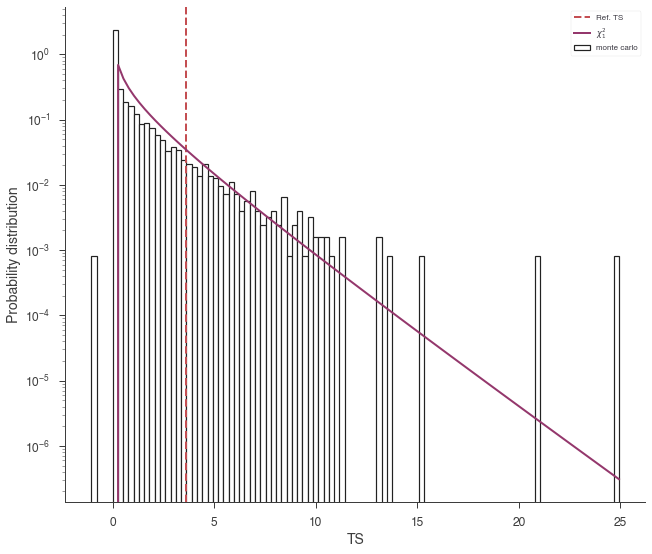The curve is slightly higher than we expect. Let’s rescale the curve by 1/2:

:

lrt.plot_TS_distribution(scale=0.5,bins=100,ec='k',fc='white',lw=1.2);
plt.legend()

:

<matplotlib.legend.Legend at 0x13e533310>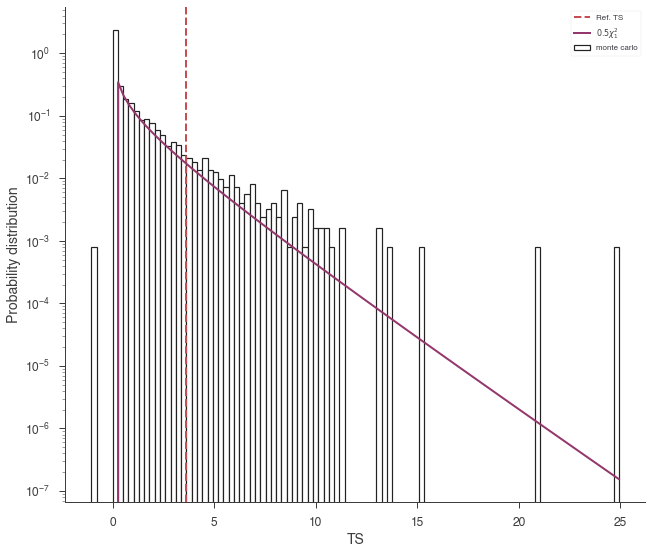Thus, we see that 3ML provides an automatic, and possibly efficient way to avoid the nasty problems of the LRT.

Both the GoodnessOfFit and LikelihoodRatioTest classes internally handle the generation of synthetic datasets. All current plugins have the ability to generate synthetic datasets based off their internal properties such as their background spectra and instrument responses.